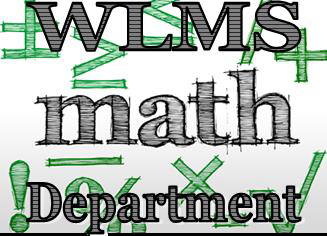## MathematicsStudents at Wolf Lake Middle get to experience learning math in an engaging, and collaborative manner. Math teachers guide students through lessons by incorporating college and career readiness standards. This student-centered, cooperative learning model teaches students to use each other, as well as their teacher, to increase their academic success. The Standards for Mathematical Practice apply throughout all courses and help to ensure that students experience mathematics as a coherent, useful, and logical subject that makes use of their ability to make sense of problem situations.

Students enrolled in Grade 6 Math discover how to (1) connect ratio and rate to whole number multiplication and division and use concepts of ratio and rate to solve problems; (2) divide fractions and extend the notion of number to the system of rational numbers, which includes negative numbers; (3) write, interpret, and use expressions and equations; and (4) think statistically. Students in Grade 6 Math also build on their work with area in elementary school by reasoning about relationships among shapes to determine area, surface area, and volume. They find areas and volumes by decomposing shapes and solids, rearranging or removing pieces, and relating the shapes to rectangles. Students discuss, develop, and justify formulas for areas of triangles and parallelograms. They prepare for work on scale drawings and constructions in Grade 7 by drawing polygons in the coordinate plane.

Grade 7 Math students (1) develop understanding of and applying proportional relationships; (2) develop understanding of operations with rational numbers and working with expressions and linear equations; (3) solve problems involving scale drawings and informal geometric constructions, and work with two- and three-dimensional shapes to solve problems involving area, surface area, and volume; and (4) draw inferences about populations based on samples.

Students in Pre-Algebra concentrate on (1) formulating and reasoning about expressions and equations, including modeling an association in bivariate data with a linear equation, and solving linear equations and systems of linear equations; (2) grasping the concept of a function and using functions to describe quantitative relationships; (3) analyzing two- and three-dimensional space and figures using distance, angle, similarity, and congruence, and understanding and applying the Pythagorean Theorem.

Algebra I students have the opportunity to earn a high school credit while formalizing and extending the mathematics that were learned in earlier grades. The critical areas of this course deepen and extend understanding of linear and exponential relationships by contrasting them with each other and by applying linear models to data that exhibit a linear trend, and students engage in methods for analyzing, solving, and using quadratic functions. The Standards for Mathematical Practice apply throughout this course and help to ensure that students experience mathematics as a coherent, useful, and logical subject that makes use of their ability to make sense of problem situations.

Geometry students earn an additional high school credit and move on to formalize and extend geometric experiences from the middle grades courses. Students explore more complex geometric situations and deepen their explanations of geometric relationships, moving towards formal mathematical arguments. Important differences exist between this Geometry course and the historical approach taken in Geometry classes. For example, transformations are emphasized early in this course.